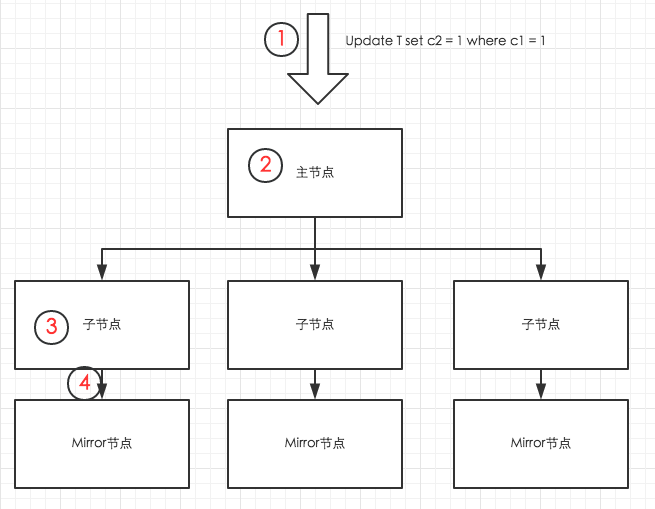# 数据库内核月报 － 2017 / 05

## 简单更新过程1. 用户把Update的SQL请求发送到主节点；

2. 主节点发起分布式事务，并对被Update的表加锁（HybridDB不允许并行的Update同一张表），然后把更新请求分发到对应的子节点。

3. 子节点通过索引扫描，定位到要更新的数据，并更新数据。对于列存表，更新逻辑其实就是删除旧的数据行，并在表的尾端写入新的数据行。（列存表）被更新的数据页面会写入内存缓存区，对应的表文件长度的变化（因为尾端写入了数据，所以数据表对应的文件长度增大了）会写入日志（xlog文件）。

4. 在Update命令结束前，内存中的被更新的数据页面和xlog日志，都要同步到Mirror节点。同步完成后，主节点结束分布式事务，返回用户执行成功的消息。

• 尽量把更新放到一个SQL语句，减少语句解析、节点通信、数据同步等开销；

• 尽量把更新放到一个事务，避免不必要的事务开销。

## 批量Update

``````create table target_table(c1 int, c2 int, primary key (c1));

insert into target_table select generate_series(1, 10000000);

``````

``````create table source_table(c1 int, c2 int);

insert into source_table select generate_series(1, 100), generate_series(1,100);

``````

source_table数据准备好后，执行下面的`update set … from … where ..`语句，即可实现批量的Update。注意，为了最大限度的使用到索引，在执行Update前，要使用`set opitimzer=on`启用ORCA优化器（如果不启用ORCA优化器，则需要执行`set enable_nestloop = on`才能使用到索引）。

``````
set optimizer=on;

update target_table set c2 = source_table.c2 from source_table where target_table.c1= source_table.c1;
``````

``````=> explain update target_table set c2 = source_table.c2 from source_table where target_table.c1= source_table.c1;
QUERY PLAN
-----------------------------------------------------------------------------------------------------------------------------
Update  (cost=0.00..586.10 rows=25 width=1)
->  Result  (cost=0.00..581.02 rows=50 width=26)
->  Redistribute Motion 4:4  (slice1; segments: 4)  (cost=0.00..581.02 rows=50 width=22)
Hash Key: public.target_table.c1
->  Assert  (cost=0.00..581.01 rows=50 width=22)
Assert Cond: NOT public.target_table.c1 IS NULL
->  Split  (cost=0.00..581.01 rows=50 width=22)
->  Nested Loop  (cost=0.00..581.01 rows=25 width=18)
Join Filter: true
->  Table Scan on source_table  (cost=0.00..431.00 rows=25 width=8)
->  Index Scan using target_table_pkey on target_table  (cost=0.00..150.01 rows=1 width=14)
Index Cond: public.target_table.c1 = source_table.c1
``````

``````postgres=> insert into source_table select generate_series(1, 1000), generate_series(1,1000);
INSERT 0 1000
postgres=> analyze source_table;
ANALYZE
postgres=> explain update target_table set c2 = source_table.c2 from source_table where target_table.c1= source_table.c1;
QUERY PLAN
------------------------------------------------------------------------------------------------------
Update  (cost=0.00..1485.82 rows=275 width=1)
->  Result  (cost=0.00..1429.96 rows=550 width=26)
->  Assert  (cost=0.00..1429.94 rows=550 width=22)
Assert Cond: NOT public.target_table.c1 IS NULL
->  Split  (cost=0.00..1429.93 rows=550 width=22)
->  Hash Join  (cost=0.00..1429.92 rows=275 width=18)
Hash Cond: public.target_table.c1 = source_table.c1
->  Table Scan on target_table  (cost=0.00..477.76 rows=2500659 width=14)
->  Hash  (cost=431.01..431.01 rows=275 width=8)
->  Table Scan on source_table  (cost=0.00..431.01 rows=275 width=8)

``````

## 批量Delete

``````delete from target_table using source_table where target_table.c1 = source_table.c1;
``````

``````explain delete from target_table using source_table where target_table.c1 = source_table.c1;
QUERY PLAN
-----------------------------------------------------------------------------------------------------
Delete (slice0; segments: 4)  (rows=50 width=10)
->  Nested Loop  (cost=0.00..41124.40 rows=50 width=10)
->  Seq Scan on source_table  (cost=0.00..6.00 rows=50 width=4)
->  Index Scan using target_table_pkey on target_table  (cost=0.00..205.58 rows=1 width=14)
Index Cond: target_table.c1 = source_table.c1
``````

## 利用Delete + Insert做数据合并

``````set optimizer=on;

delete from target_table using source_table where target_table.c1 = source_table.c1;

insert into target_table select * from source_table;

``````

## 利用Values()表达式做实时更新

``````update target_table set c2 = t.c2 from (values(1,1),(2,2),(3,3),…(2000,2000)) as t(c1,c2) where target_table.c1=t.c1

delete from target_table using (values(1,1),(2,2),(3,3),…(2000,2000)) as t(c1,c2) where target_table.c1 = t.c1
``````